# Fourier Transform Notes

The following are my notes from a talk I gave at OSCON 2013. Here is the Github source of these Jupyter/IPython notebooks: https://github.com/calebmadrigal/FourierTalkOSCON

# Sound Analysis with the Fourier Transform and Python

## Intro

1. Welcome
2. Format of talk
• Everything is in iPython Notebook (on Github)
• You don't need to take notes
• Please save questions for the end
3. Why this is interesting
• Sound processing is big - natural human-machine interfaces (e.g. Siri)
• Noise reduction, Compression, feature extraction (e.g. speech)
• Understanding our universe better (Superposition, Harmonics, Sound timbre)

## Overview

• The Nature of Waves
• The Fourier Transform
• Fast Fourier Transform (FFT) in Python
• Audio analysis

In :
%matplotlib inline
import matplotlib.pyplot as plt
import numpy as np
import scipy

# Graphing helper function
def setup_graph(title='', x_label='', y_label='', fig_size=None):
fig = plt.figure()
if fig_size != None:
fig.set_size_inches(fig_size, fig_size)
ax.set_title(title)
ax.set_xlabel(x_label)
ax.set_ylabel(y_label)


# The Nature of Waves

We're mainly going to talk about sound waves, but many of these things will apply to other types of waves.

## How do we represent waves?

In :
freq = 1 #hz - cycles per second
amplitude = 3
time_to_plot = 2 # second
sample_rate = 100 # samples per second
num_samples = sample_rate * time_to_plot

t = np.linspace(0, time_to_plot, num_samples)
signal = [amplitude * np.sin(freq * i * 2*np.pi) for i in t] # Explain the 2*pi

setup_graph(x_label='time (in seconds)', y_label='amplitude', title='time domain')
plt.plot(t, signal)

Out:
[<matplotlib.lines.Line2D at 0x10f2809e8>]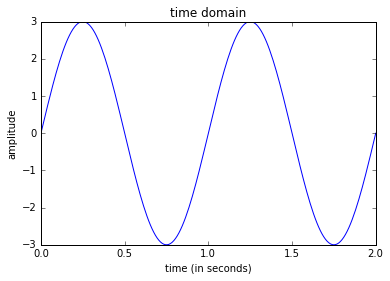## In the context of sound, what would a wave like this represent?

• Answer: Changes in air pressure
• When the graph is above x=0, the pressure of the air is more than "normal" (air is moving toward you)
• When the graph is below x=0, the pressure of the air is less than "normal" (air is moving away from you)

# Example of a real sound wave¶

In :
import scipy.io.wavfile

time_array = np.arange(0, len(input_signal)/sample_rate, 1/sample_rate)
setup_graph(title='Ah vowel sound', x_label='time (in seconds)', y_label='amplitude', fig_size=(14,7))
_ = plt.plot(time_array[0:4000], input_signal[0:4000])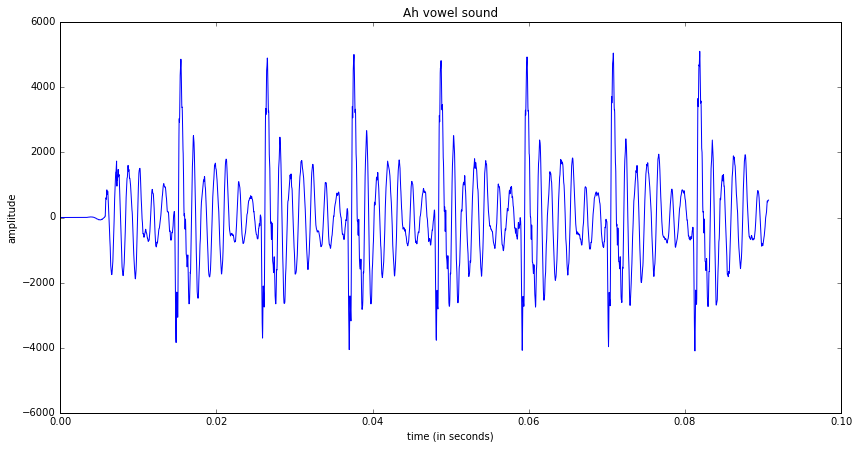# The Superposition Principle

• If you add a bunch of waves together, it forms one wave.
• Hearing me talk while knocking on the desk

# Example

In :
# Two subplots, the axes array is 1-d
x = np.linspace(0, 2 * np.pi, 100)
y1 = 5 * np.sin(x)
y2 = 0 * np.sin(2*x)
y3 = 3 * np.sin(3*x)
y4 = 2 * np.sin(4*x)

f, axarr = plt.subplots(4, sharex=True, sharey=True)
f.set_size_inches(12,6)
axarr.plot(x, y1)
axarr.plot(x, y2)
axarr.plot(x, y3)
axarr.plot(x, y4)
_ = plt.show()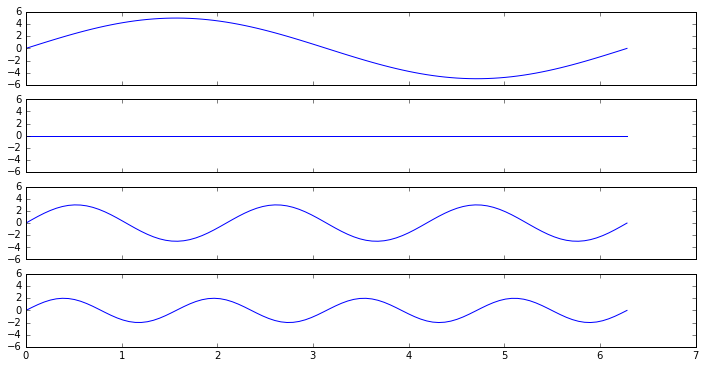In :
setup_graph(x_label='time', y_label='amplitude', title='y1+y2+y3+y4', fig_size=(12,6))
convoluted_wave = y1 + y2 + y3 + y4
_ = plt.plot(x, convoluted_wave)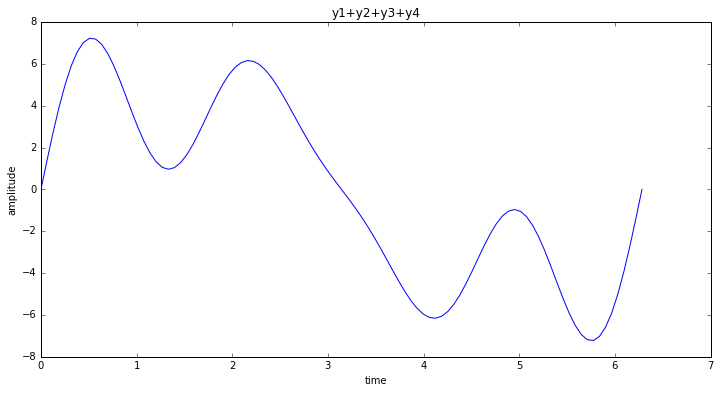# Wave Interference Example

### This is how noise-canceling headphones work

In :
y5 = np.sin(3 * x)
y6 = -1 * np.sin(3 * x)

f, axarr = plt.subplots(3, sharex=True, sharey=True)
f.set_size_inches(12,6)
axarr.plot(x, y5, 'b')
axarr.plot(x, y6, 'g')
axarr.plot(x, y5 + y6, 'r')
_ = plt.show()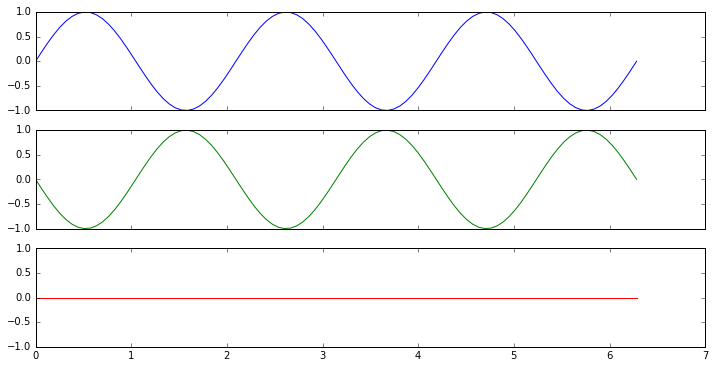In [None]:

In :
# Graphing helper function
def setup_graph(title='', x_label='', y_label='', fig_size=None):
fig = plt.figure()
if fig_size != None:
fig.set_size_inches(fig_size, fig_size)
ax.set_title(title)
ax.set_xlabel(x_label)
ax.set_ylabel(y_label)



# Fourier Transform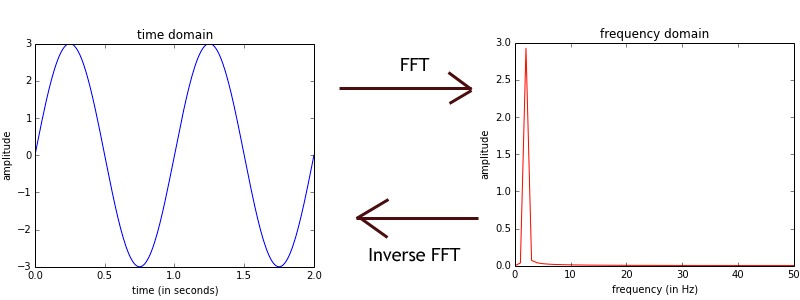Time to Frequency Domain

## The Fourier Transform is like a prism (not the NSA one)Prism

## Fourier Transform Definition

$G(f) = \int_{-\infty}^\infty g(t) e^{-i 2 \pi f t} dt$

For our purposes, we will just be using the discrete version...

## Discrete Fourier Transform (DFT) Definition

$G(\frac{n}{N}) = \sum_{k=0}^{N-1} g(k) e^{-i 2 \pi k \frac{n}{N} }$

Meaning:

• $N$ is the total number of samples
• $g(k)$ is the kth sample for the time-domain function (i.e. the DFT input)
• $G(\frac{n}{N})$ is the output of the DFT for the frequency that is $\frac{n}{N}$ cycles per sample; so to get the frequency, you have to multiply $n/N$ by the sample rate.

# How to represent waves¶

In :
%matplotlib inline
import matplotlib.pyplot as plt
import numpy as np
import scipy

freq = 1 #hz - cycles per second
amplitude = 3
time_to_plot = 2 # second
sample_rate = 100 # samples per second
num_samples = sample_rate * time_to_plot

t = np.linspace(0, time_to_plot, num_samples)
signal = [amplitude * np.sin(freq * i * 2*np.pi) for i in t] # Explain the 2*pi


### Why the 2*pi?

• If we want a wave which completes 1 cycle per second, so sine must come back to the same position on a circle as the starting point
• So one full rotation about a circle - $2 \pi$ (in radians)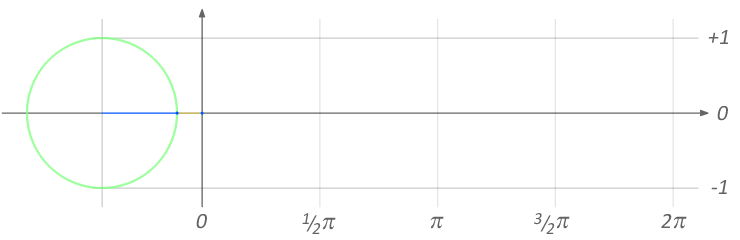sine_curve

# Plot the wave¶

In :
setup_graph(x_label='time (in seconds)', y_label='amplitude', title='time domain')
plt.plot(t, signal)

Out:
[<matplotlib.lines.Line2D at 0x10f277748>]# Convert to the Frequency Domain

In :
fft_output = np.fft.rfft(signal)
magnitude_only = [np.sqrt(i.real**2 + i.imag**2)/len(fft_output) for i in fft_output]
frequencies = [(i*1.0/num_samples)*sample_rate for i in range(num_samples//2+1)]

In :
setup_graph(x_label='frequency (in Hz)', y_label='amplitude', title='frequency domain')
plt.plot(frequencies, magnitude_only, 'r')

Out:
[<matplotlib.lines.Line2D at 0x10eec6160>]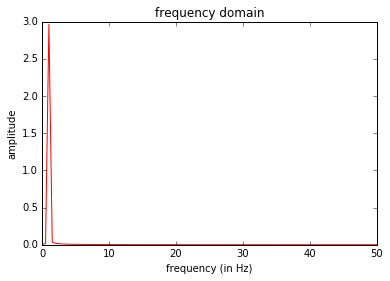## Question: So what does the Fourier Transform give us?

• The amplitudes of simple sine waves
• Their starting position - phase (we won't get into this part much)

## Question: what sine wave frequencies are used?

• Answer: This is determined by how many samples are provided to the Fourier Transform
• Frequencies range from 0 to (number of samples) / 2
• Example: If your sample rate is 100Hz, and you give the FFT 100 samples, the FFT will return the amplitude of the components with frequencies 0 to 50Hz.
In [None]:

In :
%matplotlib inline
import matplotlib.pyplot as plt
import numpy as np
import scipy

# Graphing helper function
def setup_graph(title='', x_label='', y_label='', fig_size=None):
fig = plt.figure()
if fig_size != None:
fig.set_size_inches(fig_size, fig_size)
ax.set_title(title)
ax.set_xlabel(x_label)
ax.set_ylabel(y_label)


# Wave Deconvolution - Multiplication and Addition

• So a complex wave is made of simple waves.
• How can you break a complex wave into it's simple component waves?

### Answer: Multiplying a wave by another wave of frequency 100Hz cancels out all of the other component waves and leaves only the 100Hz component (kind of).

In :
t = np.linspace(0, 3, 200)
freq_1hz_amp_10 = 10 * np.sin(1 * 2*np.pi*t)
freq_3hz_amp_5 =   5 * np.sin(3 * 2*np.pi*t)
complex_wave = freq_1hz_amp_10 + freq_3hz_amp_5

setup_graph(x_label='time (in seconds)', y_label='amplitude', title='original wave', fig_size=(12,6))
_ = plt.plot(t, complex_wave)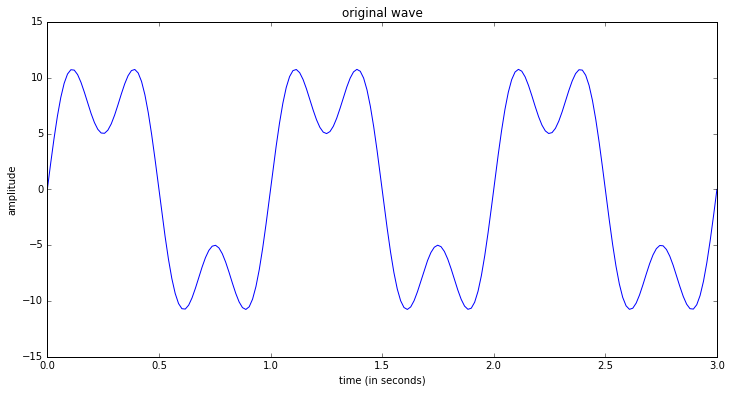# Multiply complex wave by 1Hz wave¶

In :
freq_1hz = np.sin(1 * 2*np.pi*t)
setup_graph(x_label='time (in seconds)', y_label='amplitude', title='original wave * 1Hz wave', fig_size=(12,6))
_ = plt.plot(t, complex_wave * freq_1hz)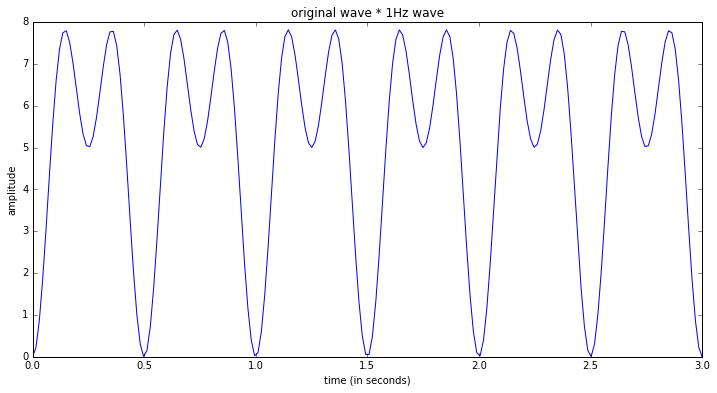In :
sum(complex_wave*freq_1hz)

Out:
994.99999999999977

In :
print("Amplitude of 1hz component: ", sum(complex_wave*freq_1hz) * 2.0 * 1.0/len(complex_wave))

Amplitude of 1hz component:  9.95



# Multiply complex wave by 3Hz wave

Notice that more of the graph is above the x-axis then below it.

In :
freq_3hz = np.sin(3 * 2*np.pi*t)
setup_graph(x_label='time (in seconds)', y_label='amplitude', title='complex wave * 3Hz wave', fig_size=(12,6))
_ = plt.plot(t, complex_wave * freq_3hz)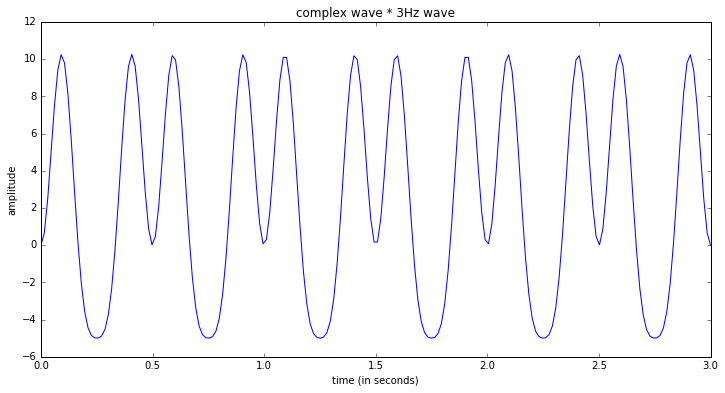In :
sum(complex_wave*freq_3hz)

Out:
497.5

In :
print("Amplitude of 3hz component: ", sum(complex_wave*freq_3hz) * 2.0/len(complex_wave))

Amplitude of 3hz component:  4.975



# Multiply complex wave by 2Hz wave

Notice that an equal amount of the graph is above the x-axis as below it.

In :
freq_2hz = np.sin(2 * 2*np.pi*t)
setup_graph(x_label='time (in seconds)', y_label='amplitude', title='complex wave * 2Hz wave', fig_size=(12,6))
_ = plt.plot(t, complex_wave * freq_2hz)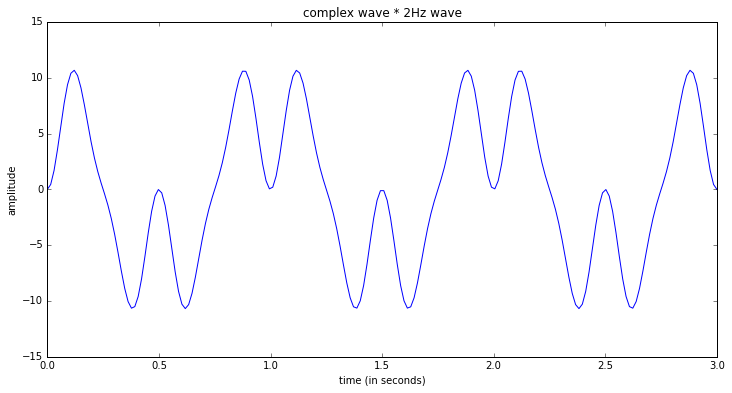In :
sum(complex_wave*freq_2hz)

Out:
1.4549472737712679e-13

In :
# Very close to 0
print("Amplitude of 3hz component: ", sum(complex_wave*freq_2hz) * 2.0/len(complex_wave))

Amplitude of 3hz component:  1.45494727377e-15


In :
# Same with 4Hz - close to 0
freq_4hz = np.sin(4 * 2*np.pi*t)
sum(complex_wave*freq_4hz)

Out:
-5.0848214527832113e-14


# So how does this work?

The summation of complex wave multiplied by simple wave of a given frequency leaves us with the "power" of that simple wave.

In :
%matplotlib inline
import matplotlib.pyplot as plt
import numpy as np
import scipy

# Graphing helper function
def setup_graph(title='', x_label='', y_label='', fig_size=None):
fig = plt.figure()
if fig_size != None:
fig.set_size_inches(fig_size, fig_size)
ax.set_title(title)
ax.set_xlabel(x_label)
ax.set_ylabel(y_label)


# Euler's Formula

$e^{ix} = \cos(x) + i \sin(x)$

So raising $e^{ix}$ produces rotation (in the Complex plane)

$e^{i \pi} + 1 = 0$

• At $x = \pi$, $\sin(\pi) = 0$, and $\cos(\pi) = -1$
• So $e^{i \pi} = -1 + 0$
• Move the 1 over: $e^{i \pi} + 1 = 0$

## Complex Plane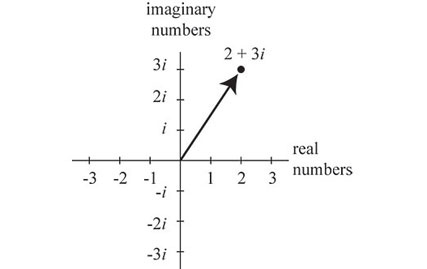Complex Plane

In :
# Generate some complex numbers, and convert them to (x,y) coordinates
complex_points = [np.e**(1j * i) for i in np.linspace(0, 2*np.pi, 16)]
real_parts = [z.real for z in complex_points]
imag_parts = [z.imag for z in complex_points]

# Matplotlib code to draw graph
fig = plt.figure()
fig.set_size_inches(6, 6)
ax.spines['left'].set_position('center')
ax.spines['bottom'].set_position('center')
ax.spines['top'].set_color('none')
ax.spines['right'].set_color('none')
ax.set_xlim((-1.5,1.5))
ax.set_xticks(np.linspace(-1.5,1.5,4))
ax.set_ylim((-1.5,1.5))
ax.set_yticks(np.linspace(-1.5,1.5,4))
ax.annotate("{0} + {1}i".format(real_parts, imag_parts), xy=(real_parts, \
imag_parts), xytext=(1,1), arrowprops=dict(facecolor='black', shrink=0.08))

_ = plt.plot(real_parts, imag_parts, 'go')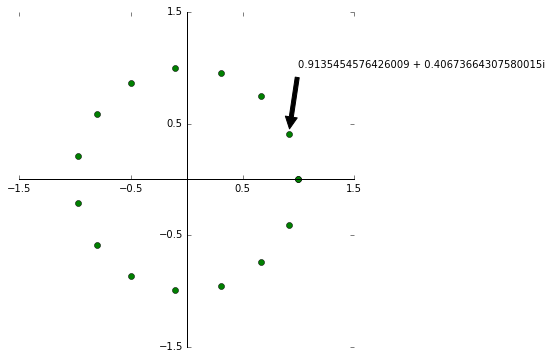### Going around a circle produces sine and cosine wavessine_curve

# Circles produce sin (and cos) waves¶

In :
t = np.linspace(0, 3 * 2*np.pi, 100)
e_func = [np.e**(1j * i) for i in t]

In :
setup_graph(title='real part (cosine) of e^ix', x_label='time', y_label='amplitude of real part', fig_size=(12,6))
_ = plt.plot(t, [i.real for i in e_func])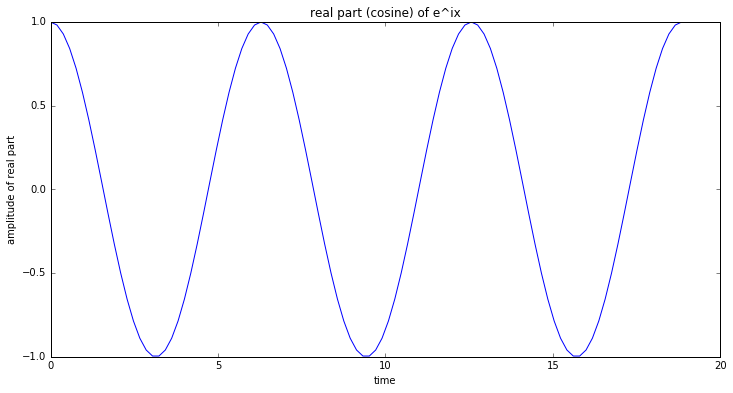In :
setup_graph(title='imaginary part (sin) of e^ix', x_label='time', y_label='amplitude of imaginary part', fig_size=(12,6))
_ = plt.plot(t, [i.imag for i in e_func])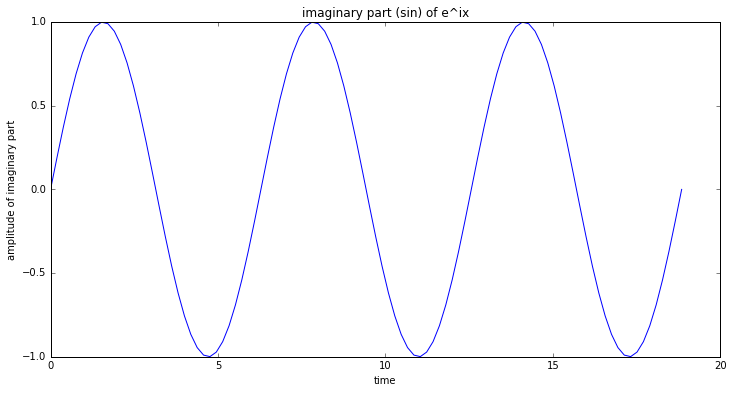In [None]:

In :
%matplotlib inline
import matplotlib.pyplot as plt
import numpy as np
import scipy

# Graphing helper function
def setup_graph(title='', x_label='', y_label='', fig_size=None):
fig = plt.figure()
if fig_size != None:
fig.set_size_inches(fig_size, fig_size)
ax.set_title(title)
ax.set_xlabel(x_label)
ax.set_ylabel(y_label)


# Sampling a test function

Wave with:

• Frequency of 1 Hz
• Amplitude of 5

We will sample:

• At 10 samples per second
• For 3 seconds
• So a total of 30 samples
In :
sample_rate = 10 # samples per sec
total_sampling_time = 3
num_samples = sample_rate * total_sampling_time

t = np.linspace(0, total_sampling_time, num_samples)

# between x = 0 and x = 1, a complete revolution (2 pi) has been made, so this is
# a 1 Hz signal with an amplitude of 5
frequency_in_hz = 1
wave_amplitude = 5
f = lambda x: wave_amplitude * np.sin(frequency_in_hz * 2*np.pi*x)

sampled_f = [f(i) for i in t]

In :
setup_graph(title='time domain', x_label='time (in seconds)', y_label='amplitude', fig_size=(12,6))
_ = plt.plot(t, sampled_f)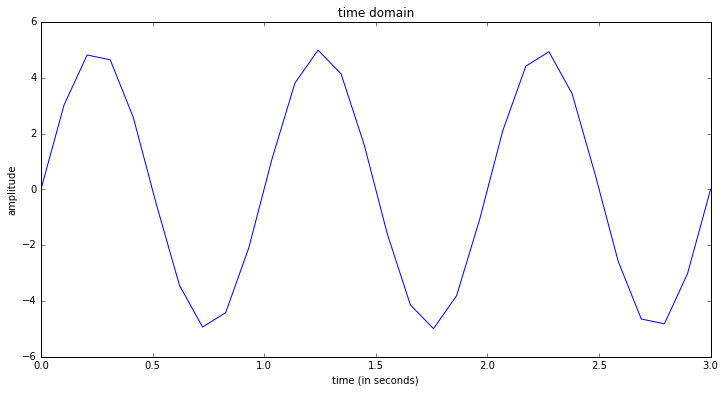# Take the fft¶

In :
fft_output = np.fft.fft(sampled_f)

In :
setup_graph(title='FFT output', x_label='FFT bins', y_label='amplitude', fig_size=(12,6))
_ = plt.plot(fft_output)

/usr/local/lib/python3.5/site-packages/numpy/core/numeric.py:482: ComplexWarning: Casting complex values to real discards the imaginary part
return array(a, dtype, copy=False, order=order)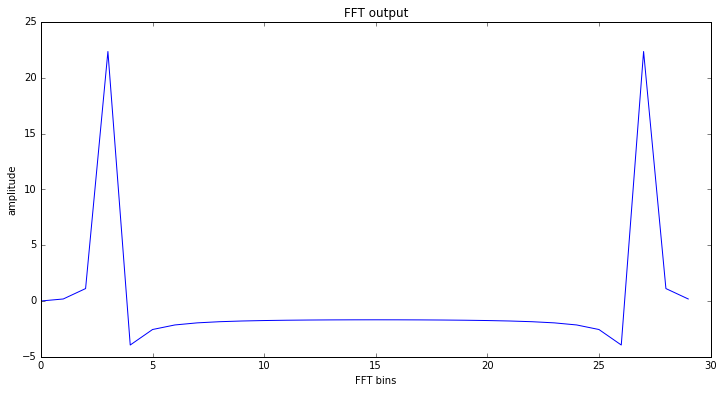# Why is it symmetric?

• Because it is a complex-input fourier transform, and for real input, the 2nd half will always be a mirror image.
• For real-valued input, the fft output is always symmetric.
• Since we are only dealing with real input, let's just use a real-input version of the fft.
In :
rfft_output = np.fft.rfft(sampled_f)

In :
setup_graph(title='rFFT output', x_label='frequency (in Hz)', y_label='amplitude', fig_size=(12,6))
_ = plt.plot(rfft_output)

/usr/local/lib/python3.5/site-packages/numpy/core/numeric.py:482: ComplexWarning: Casting complex values to real discards the imaginary part
return array(a, dtype, copy=False, order=order)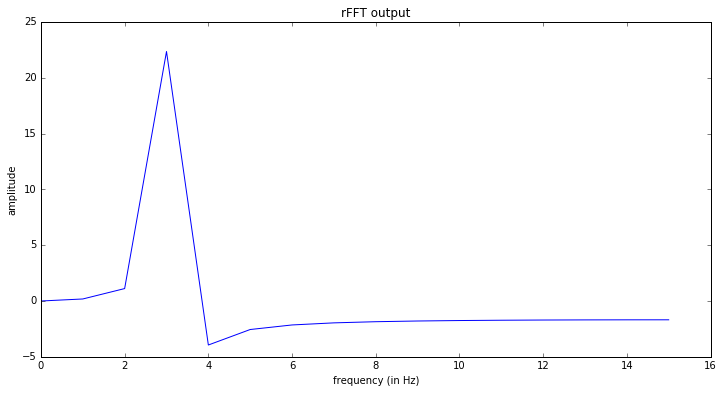# Get the x-axis labels correct

## We want the x-axis to represent frequency

In our DFT equation $G(\frac{n}{N}) = \sum_{k=0}^{N-1} g(k) e^{-i 2 \pi k \frac{n}{N} }$

• $G(\frac{n}{N})$ means the DFT output for the frequency which goes through $\frac{n}{N}$ cycles per sample.
• And we take frequencies from 0 to (the total number of samples divided by 2)
In :
# Getting frequencies on x-axis right
rfreqs = [(i*1.0/num_samples)*sample_rate for i in range(num_samples//2+1)]


### Frequencies range from 0 to the Nyquist Frequency (sample rate / 2)¶

In :
# So you can see, our frequencies go from 0 to 5Hz.  5 is half of the sample rate,
# which is what it should be (Nyquist Frequency).
rfreqs

Out:
[0.0,
0.3333333333333333,
0.6666666666666666,
1.0,
1.3333333333333333,
1.6666666666666665,
2.0,
2.3333333333333335,
2.6666666666666665,
3.0,
3.333333333333333,
3.6666666666666665,
4.0,
4.333333333333334,
4.666666666666667,
5.0]

In :
setup_graph(title='Corrected rFFT Output', x_label='frequency (in Hz)', y_label='amplitude', fig_size=(12,6))
_ = plt.plot(rfreqs, rfft_output)

/usr/local/lib/python3.5/site-packages/numpy/core/numeric.py:482: ComplexWarning: Casting complex values to real discards the imaginary part
return array(a, dtype, copy=False, order=order)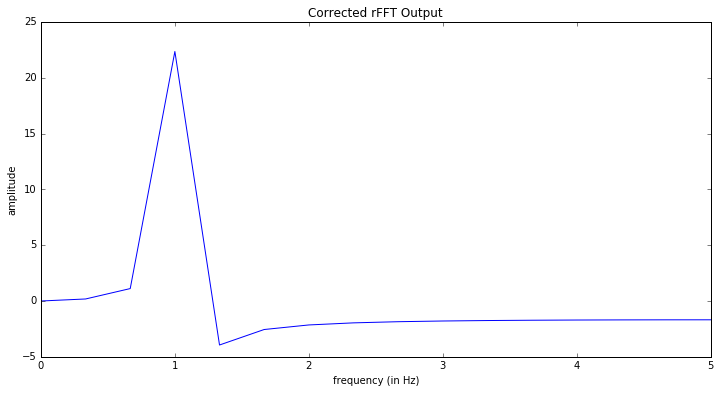# Getting y-axis labels correct

## We want the y-axis to represent magnitude

We are actually getting negative values, and it looks like our amplitude is way larger than what it should be (which is 5).

The magnitude of each component is:

$magnitude(i) = \frac{\sqrt{i.real^2 + i.imag^2}}{N}$

In :
rfft_mag = [np.sqrt(i.real**2 + i.imag**2)/len(rfft_output) for i in rfft_output]

In :
setup_graph(title='Corrected rFFT Output', x_label='frequency (in Hz)', y_label='magnitude', fig_size=(12,6))
_ = plt.plot(rfreqs, rfft_mag)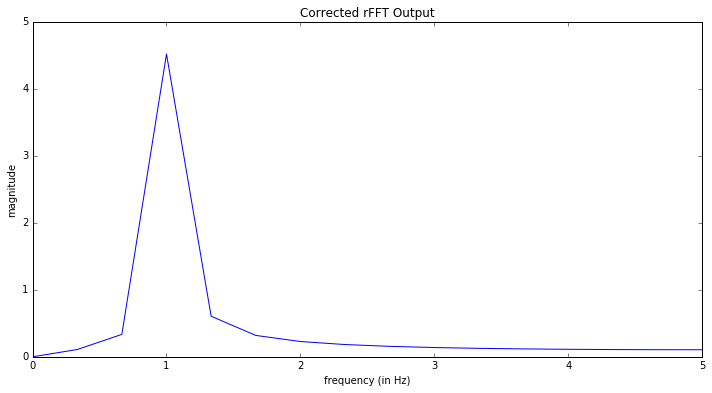# Inverse FFT

We can take the output of the FFT and perform an Inverse FFT to get back to our original wave (using the Inverse Real FFT - irfft).

In :
irfft_output = np.fft.irfft(rfft_output)

In :
setup_graph(title='Inverse rFFT Output', x_label='time (in seconds)', y_label='amplitude', fig_size=(12,6))
_ = plt.plot(t, irfft_output)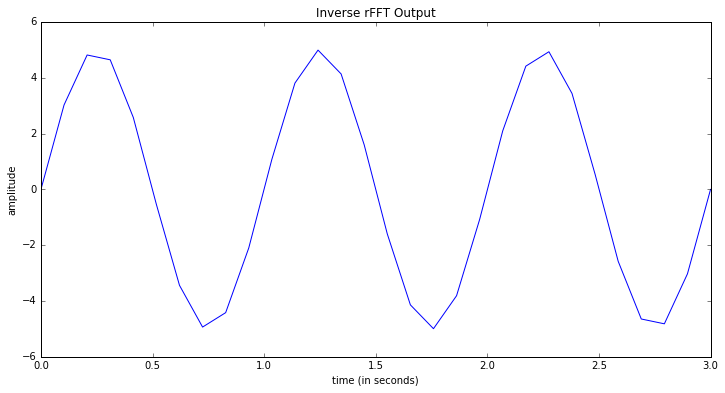In [None]:

In :
%matplotlib inline
import matplotlib.pyplot as plt
import numpy as np
import scipy
import scipy.io.wavfile
import IPython

def setup_graph(title='', x_label='', y_label='', fig_size=None):
fig = plt.figure()
if fig_size != None:
fig.set_size_inches(fig_size, fig_size)
ax.set_title(title)
ax.set_xlabel(x_label)
ax.set_ylabel(y_label)


# Seeing sound!¶

In :
# NOTE: This is only works with 1 channel (mono).  To record a mono audio sample,
# you can use this command: rec -r 44100 -c 1 -b 16 test.wav
time_array = np.arange(0, len(input_signal)/sample_rate, 1/sample_rate)

In :
setup_graph(title='Ah vowel sound', x_label='time (in seconds)', y_label='amplitude', fig_size=(14,7))
_ = plt.plot(time_array[0:4000], input_signal[0:4000])In :
#IPython.display.Audio("audio_files/vowel_ah.wav")

In :
fft_out = np.fft.rfft(input_signal)
fft_mag = [np.sqrt(i.real**2 + i.imag**2)/len(fft_out) for i in fft_out]
num_samples = len(input_signal)
rfreqs = [(i*1.0/num_samples)*sample_rate for i in range(num_samples//2+1)]

setup_graph(title='FFT of Ah Vowel (first 5000)', x_label='FFT Bins', y_label='magnitude', fig_size=(14,7))
_ = plt.plot(rfreqs[0:5000], fft_mag[0:5000])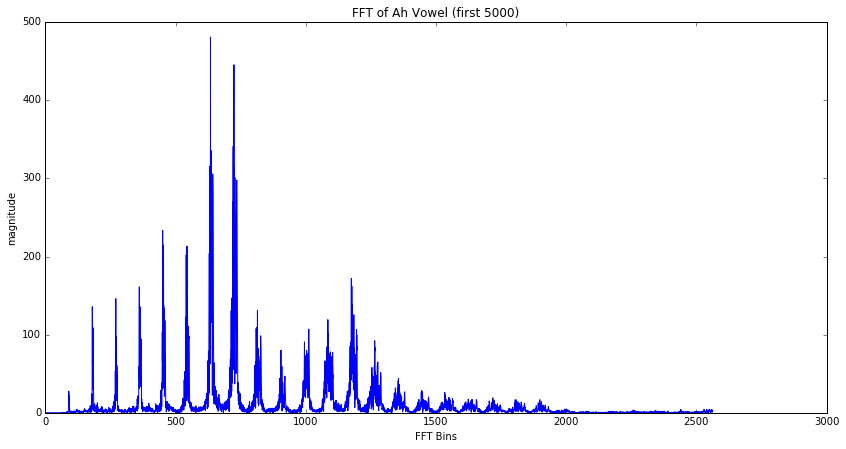• Ratio of harmonics = timbre = This is what makes different people's voices sound different (and different from violins)
• Even if they are singing the same note, and the same volume
• Possible application: synthesizing new sounds (with different harmonic profiles)
• Example: If you changed the ratio of the harmonics, you could make your voice sound like something else (Darth Vader?)

# Spectrogram (FFT over time)

### Axes

• x-axis: time
• y-axis: frequency
• z-axis (color): strength of each frequency

### See the Harmonics!

In :
(doremi_sample_rate, doremi) = scipy.io.wavfile.read("audio_files/do-re-mi.wav")

In :
setup_graph(title='Spectrogram of diatonic scale (44100Hz sample rate)', x_label='time (in seconds)', y_label='frequency', fig_size=(14,8))
_ = plt.specgram(doremi, Fs=doremi_sample_rate)